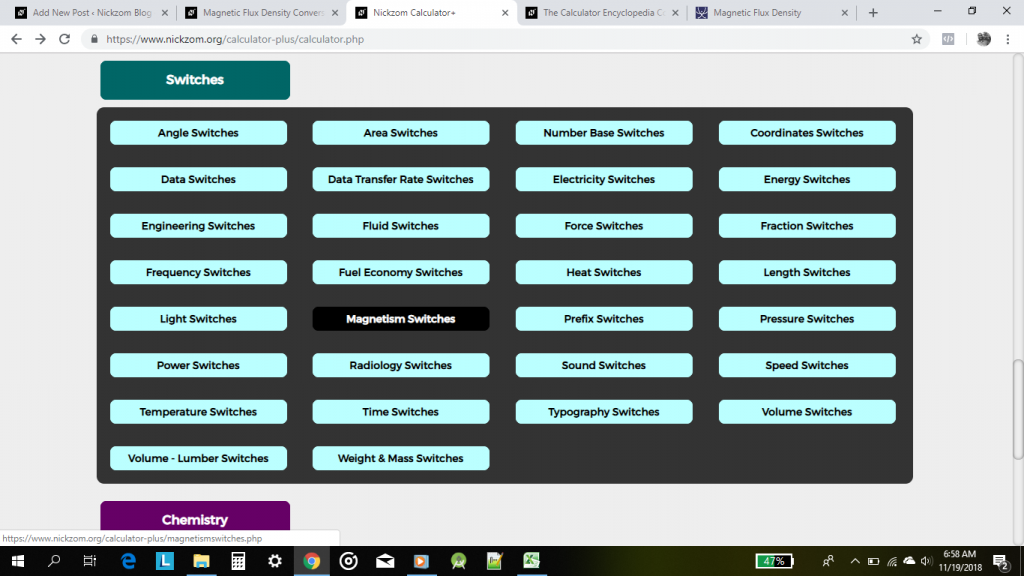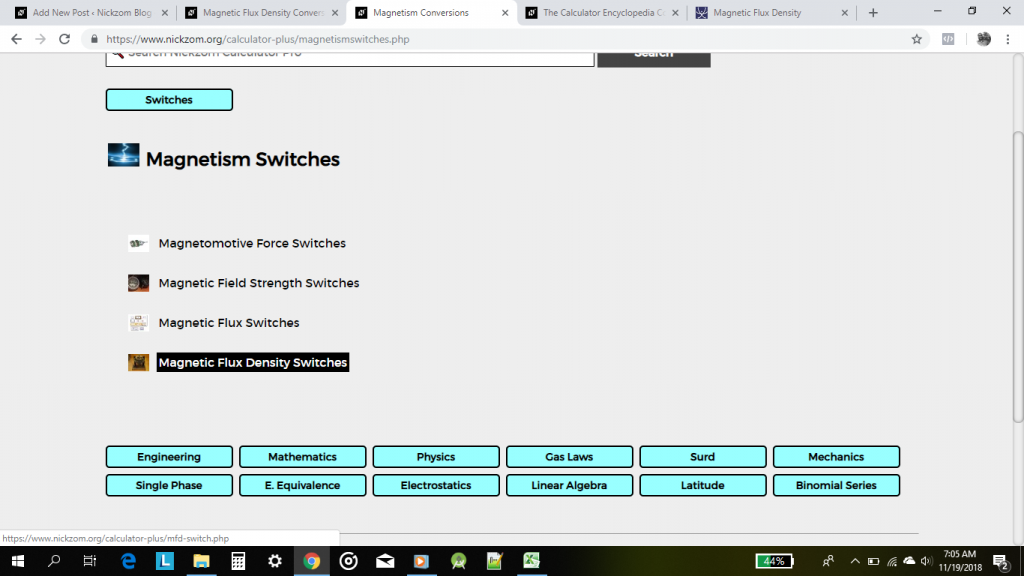## The Calculator Encyclopedia Converts Magnetic Flux Density Units on Magnetism Unit Conversions

According to Mini Physics,

Magnetic flux density (B) is defined as the force acting per unit current per unit length on a wire placed at right angles to the magnetic field.Nickzom Calculator converts 11 units of magnetic flux density such as:

• Tesla
• Weber/sq. meter
• Weber/sq. centimeter
• Weber/sq. inch
• Maxwell/sq. meter
• Maxwell/sq. centimeter
• Maxwell/sq. inch
• Gauss
• Lines/sq. centimeter
• Lines/sq. inch
• Gamma

On Clicking on Magnetism Switches, there are 4 major magnetism unit conversion sections such as:

• Magnetomotive Force Switches
• Magnetic Field Strength Switches
• Magnetic Flux Switches
• Magnetic Flux Density Switches